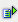The function count (range) calculates the number of numerical values within a range.

Syntax: =count(range)Example

Cell A5 = count(A1:A4)

 R / C A 1 1 2 text 3 4 3 5 2

The number of numerical values in the cells A1 to A4 is 2